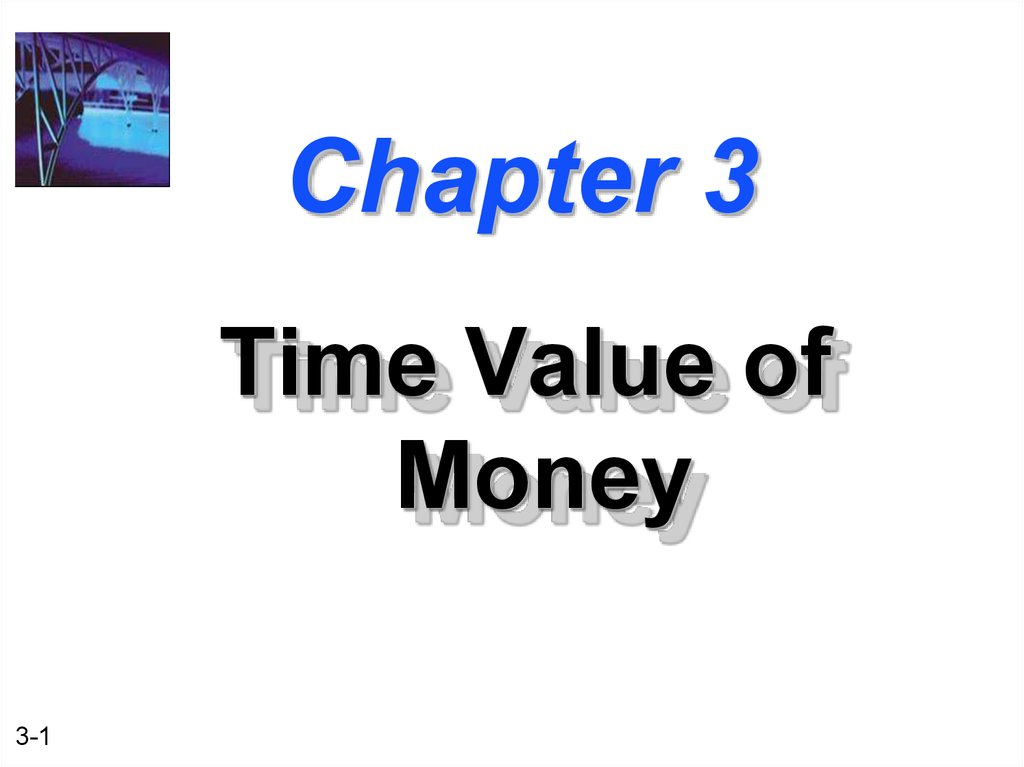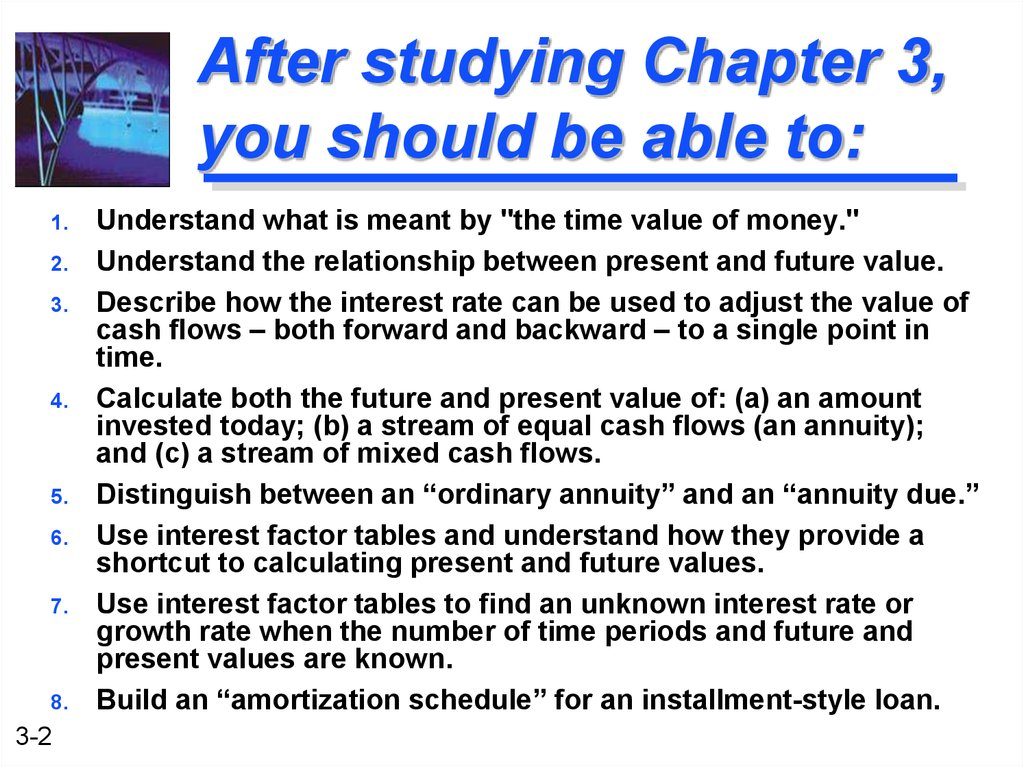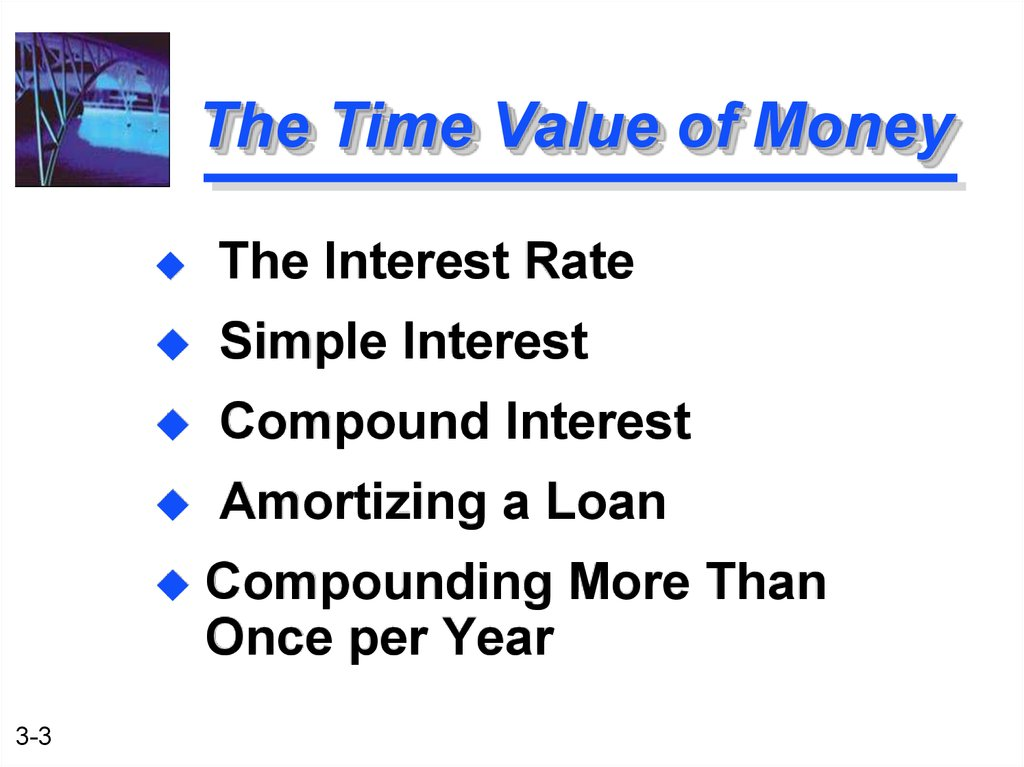# Time Value of Money

Time Value of
Money
3-1

## 2. After studying Chapter 3, you should be able to:

1.
2.
3.
4.
5.
6.
7.
8.
3-2
Understand what is meant by "the time value of money."
Understand the relationship between present and future value.
Describe how the interest rate can be used to adjust the value of
cash flows – both forward and backward – to a single point in
time.
Calculate both the future and present value of: (a) an amount
invested today; (b) a stream of equal cash flows (an annuity);
and (c) a stream of mixed cash flows.
Distinguish between an “ordinary annuity” and an “annuity due.”
Use interest factor tables and understand how they provide a
shortcut to calculating present and future values.
Use interest factor tables to find an unknown interest rate or
growth rate when the number of time periods and future and
present values are known.
Build an “amortization schedule” for an installment-style loan.

## 3. The Time Value of Money

The Interest Rate
Simple Interest
Compound Interest
Amortizing a Loan
3-3
Compounding More Than
Once per Year

## 4. The Interest Rate

Which would you prefer -- \$10,000
today or \$10,000 in 5 years?
Obviously, \$10,000 today.
You already recognize that there is
TIME VALUE TO MONEY!!
3-4

## 5. Why TIME?

Why is TIME such an important
TIME allows you the opportunity to
postpone consumption and earn
INTEREST.
3-5

## 6. Types of Interest

Simple
Interest
Interest paid (earned) on only the original
amount, or principal, borrowed (lent).
Compound
Interest
Interest paid (earned) on any previous
interest earned, as well as on the
principal borrowed (lent).
3-6

## 7. Simple Interest Formula

Formula
3-7
SI = P0(i)(n)
SI:
Simple Interest
P0:
Deposit today (t=0)
i:
Interest Rate per Period
n:
Number of Time Periods

## 8. Simple Interest Example

Assume
that you deposit \$1,000 in an
account earning 7% simple interest for
2 years. What is the accumulated
interest at the end of the 2nd year?
SI
3-8
= P0(i)(n)
= \$1,000(.07)(2)
= \$140

## 9. Simple Interest (FV)

What
is the Future Value (FV) of the
deposit?
FV
Future
= P0 + SI
= \$1,000 + \$140
= \$1,140
Value is the value at some future
time of a present amount of money, or a
series of payments, evaluated at a given
interest rate.
3-9

## 10. Simple Interest (PV)

What
is the Present Value (PV) of the
previous problem?
The Present Value is simply the
\$1,000 you originally deposited.
That is the value today!
Present
3-10
Value is the current value of a
future amount of money, or a series of
payments, evaluated at a given interest
rate.

## 11. Why Compound Interest?

Future Value (U.S. Dollars)
Future Value of a Single \$1,000 Deposit
3-11
20000
10% Simple
Interest
7% Compound
Interest
10% Compound
Interest
15000
10000
5000
0
1st Year 10th
Year
20th
Year
30th
Year

## 12. Future Value Single Deposit (Graphic)

Assume that you deposit \$1,000 at
a compound interest rate of 7% for
2 years.
0
7%
1
2
\$1,000
FV2
3-12

## 13. Future Value Single Deposit (Formula)

FV1 = P0 (1+i)1
= \$1,000 (1.07)
= \$1,070
Compound Interest
You earned \$70 interest on your \$1,000
deposit over the first year.
This is the same amount of interest you
would earn under simple interest.
3-13

## 14.

Future Value
Single Deposit (Formula)
FV1
= P0 (1+i)1
FV2
= FV1 (1+i)1
= P0 (1+i)(1+i) = \$1,000(1.07)(1.07)
= P0 (1+i)2
= \$1,000(1.07)2
= \$1,144.90
= \$1,000 (1.07)
= \$1,070
You earned an EXTRA \$4.90 in Year 2 with
compound over simple interest.
3-14

## 15. General Future Value Formula

FV1 = P0(1+i)1
FV2 = P0(1+i)2
etc.
General Future Value Formula:
FVn = P0 (1+i)n
or FVn = P0 (FVIFi,n) -- See Table I
3-15

## 16. Valuation Using Table I

FVIFi,n is found on Table I
at the end of the book.
3-16
Period
1
2
3
4
5
6%
1.060
1.124
1.191
1.262
1.338
7%
1.070
1.145
1.225
1.311
1.403
8%
1.080
1.166
1.260
1.360
1.469

## 17. Using Future Value Tables

FV2
= \$1,000 (FVIF7%,2)
= \$1,000 (1.145)
= \$1,145 [Due to Rounding]
Period
6%
7%
8%
1
1.060
1.070
1.080
2
1.124
1.166
1.145
3
1.191
1.225
1.260
4
1.262
1.311
1.360
5
1.338
1.403
1.469
3-17

## 18. Using MS Excel

=FV(rate, nper, pmt,pv)
=FV is a function used for
calculating future value
3-18
Rate= the interest rate
Nper = number of periods
Pv=the present value

## 19. Story Problem Example

Julie Miller wants to know how large her deposit
of \$10,000 today will become at a compound
annual interest rate of 10% for 5 years.
0
1
2
3
4
5
10%
\$10,000
FV5
3-19

## 20. Story Problem Solution

Calculation based on general formula:
FVn = P0 (1+i)n
FV5 = \$10,000 (1+ 0.10)5
= \$16,105.10
Calculation
based on Table I:
FV5 = \$10,000 (FVIF10%, 5)
= \$10,000 (1.611)
= \$16,110 [Due to Rounding]
3-20

## 21. Using Excel

=FV(0.1,5,,-10000)
Interest
Nper
PV
= \$16,105.10
= 10% or 0.1
=5
= -10,000 since it is an
investment, it is negative equity
3-21

Quick! How long does it take to
double \$5,000 at a compound rate
of 12% per year (approx.)?
We will use the “Rule-of-72”.
3-22

## 23. The “Rule-of-72”

Quick! How long does it take to
double \$5,000 at a compound rate
of 12% per year (approx.)?
Approx. Years to Double = 72 / i%
72 / 12% = 6 Years
[Actual Time is 6.12 Years]
3-23

## 24. Using Excel

3-24
=nper(rate, pmt,pv, fv) .
=nper(.12,, -5000,10000)
=6.11 years

## 25. Present Value Single Deposit (Graphic)

Assume that you need \$1,000 in 2 years.
Let’s examine the process to determine
how much you need to deposit today at a
discount rate of 7% compounded annually.
0
7%
1
2
\$1,000
PV0
3-25
PV1

## 26. Present Value Single Deposit (Formula)

PV0 = FV2 / (1+i)2
= FV2 / (1+i)2
0
7%
= \$1,000 / (1.07)2
= \$873.44
1
2
\$1,000
PV0
3-26

## 27. General Present Value Formula

PV0 = FV1 / (1+i)1
PV0 = FV2 / (1+i)2
etc.
General Present Value Formula:
PV0 = FVn / (1+i)n
or PV0 = FVn (PVIFi,n) -- See Table II
3-27

## 28. Valuation Using Table II

PVIFi,n is found on Table II
at the end of the book.
Period
1
2
3
4
5
3-28
6%
.943
.890
.840
.792
.747
7%
.935
.873
.816
.763
.713
8%
.926
.857
.794
.735
.681

## 29. Using Present Value Tables

PV2
3-29
= \$1,000 (PVIF7%,2)
= \$1,000 (.873)
= \$873 [Due to Rounding]
Period
6%
7%
8%
1
.943
.935
.926
2
.890
.873
.857
3
.840
.816
.794
4
.792
.763
.735
5
.747
.713
.681

## 30. Story Problem Example

Julie Miller wants to know how large of a
deposit to make so that the money will
grow to \$10,000 in 5 years at a discount
rate of 10%.
0
1
2
3
4
5
10%
\$10,000
PV0
3-30

## 31. Story Problem Solution

Calculation based on general formula:
PV0 = FVn / (1+i)n
PV0 = \$10,000 / (1+ 0.10)5
= \$6,209.21
Calculation based on Table I:
PV0 = \$10,000 (PVIF10%, 5)
= \$10,000 (.621)
= \$6,210.00 [Due to Rounding]
3-31

## 32. Types of Annuities

An
Annuity represents a series of equal
payments (or receipts) occurring over a
specified number of equidistant periods.
Ordinary
Annuity: Payments or receipts
occur at the end of each period.
Annuity
Due: Payments or receipts
occur at the beginning of each period.
3-32

## 33. Examples of Annuities

3-33
Student Loan Payments
Car Loan Payments
Mortgage Payments
Retirement Savings

## 34. Parts of an Annuity

(Ordinary Annuity)
End of
Period 1
0
Today
3-34
End of
Period 2
End of
Period 3
1
2
3
\$100
\$100
\$100
Equal Cash Flows
Each 1 Period Apart

## 35. Parts of an Annuity

(Annuity Due)
Beginning of
Period 1
0
1
2
\$100
\$100
\$100
Today
3-35
Beginning of
Period 2
Beginning of
Period 3
3
Equal Cash Flows
Each 1 Period Apart

## 36. Overview of an Ordinary Annuity -- FVA

Cash flows occur at the end of the period
0
1
2
i%
n
. . .
R
R
R
R = Periodic
Cash Flow
FVAn =
R(1+i)n-1 +
R(1+i)n-2 +
... + R(1+i)1 + R(1+i)0
3-36
FVAn
n+1

## 37. Example of an Ordinary Annuity -- FVA

Cash flows occur at the end of the period
0
1
2
3
\$1,000
\$1,000
4
7%
\$1,000
\$1,070
\$1,145
FVA3 = \$1,000(1.07)2 +
\$1,000(1.07)1 + \$1,000(1.07)0 \$3,215 = FVA3
= \$1,145 + \$1,070 + \$1,000
= \$3,215
3-37

## 38. Hint on Annuity Valuation

The future value of an ordinary
annuity can be viewed as
occurring at the end of the last
cash flow period, whereas the
future value of an annuity due
can be viewed as occurring at
the beginning of the last cash
flow period.
3-38

## 39. Valuation Using Table III

FVAn
FVA3
= R (FVIFAi%,n)
= \$1,000 (FVIFA7%,3)
= \$1,000 (3.215) = \$3,215
Period
6%
7%
8%
1
1.000
1.000
1.000
2
2.060
2.070
2.080
3
3.184
3.246
3.215
4
4.375
4.440
4.506
5
5.637
5.751
5.867
3-39

## 40. Overview View of an Annuity Due -- FVAD

Cash flows occur at the beginning of the period
0
1
2
3
R
R
R
FVADn = R(1+i)n + R(1+i)n-1 +
... + R(1+i)2 + R(1+i)1
= FVAn (1+i)
3-40
n
. . .
i%
R
n-1
R

## 41. Example of an Annuity Due -- FVAD

Cash flows occur at the beginning of the period
0
1
2
3
\$1,000
\$1,000
\$1,070
4
7%
\$1,000
\$1,145
\$1,225
2
1
\$1,000(1.07) + \$1,000(1.07)
= \$1,225 + \$1,145 + \$1,070
= \$3,440
3-41

## 42. Overview of an Ordinary Annuity -- PVA

Cash flows occur at the end of the period
0
1
2
i%
n
n+1
. . .
R
R
R
R = Periodic
Cash Flow
PVAn
PVAn = R/(1+i)1 + R/(1+i)2
+ ... + R/(1+i)n
3-42

## 43. Example of an Ordinary Annuity -- PVA

Cash flows occur at the end of the period
0
1
2
3
\$1,000
\$1,000
4
7%
\$1,000
\$934.58
\$873.44
\$816.30
\$2,624.32 = PVA3
3-43
PVA3 =
\$1,000/(1.07)1 +
\$1,000/(1.07)2 +
\$1,000/(1.07)3
= \$934.58 + \$873.44 + \$816.30
= \$2,624.32

## 44. Hint on Annuity Valuation

The present value of an ordinary
annuity can be viewed as
occurring at the beginning of the
first cash flow period, whereas
the future value of an annuity
due can be viewed as occurring
at the end of the first cash flow
period.
3-44

## 45. Valuation Using Table IV

PVAn
PVA3
= R (PVIFAi%,n)
= \$1,000 (PVIFA7%,3)
= \$1,000 (2.624) = \$2,624
Period
6%
7%
8%
1
0.943
0.935
0.926
2
1.833
1.808
1.783
3
2.673
2.577
2.624
4
3.465
3.387
3.312
5
4.212
4.100
3.993
3-45

## 46. Overview of an Annuity Due -- PVAD

Cash flows occur at the beginning of the period
0
1
2
i%
R
n-1
n
. . .
R
R
R
R: Periodic
Cash Flow
PVADn = R/(1+i)0 + R/(1+i)1 + ... + R/(1+i)n-1
= PVAn (1+i)
3-46

## 47. Example of an Annuity Due -- PVAD

Cash flows occur at the beginning of the period
0
1
2
\$1,000
\$1,000
3
7%
\$1,000.00
\$ 934.58
\$ 873.44
PVADn = \$1,000/(1.07)0 + \$1,000/(1.07)1 +
\$1,000/(1.07)2 = \$2,808.02
3-47
4

## 48. Valuation Using Table IV

= \$1,000 (2.624)(1.07) = \$2,808
Period
6%
7%
8%
1
0.943
0.935
0.926
2
1.833
1.808
1.783
3
2.673
2.577
2.624
4
3.465
3.387
3.312
5
4.212
4.100
3.993
3-48

## 49. Solving the PVAD Problem

Inputs
3
7
N
I/Y
PV
-1,000
0
PMT
FV
2,808.02
Compute
Complete the problem the same as an “ordinary annuity”
problem, except you must change the calculator setting
to “BGN” first. Don’t forget to change back!
Step 1:
Press
2nd
BGN
keys
3-49
Step 2:
Press
2nd
SET
keys
Step 3:
Press
2nd
QUIT
keys

## 50. Steps to Solve Time Value of Money Problems

2. Create a time line
3. Put cash flows and arrows on time line
4. Determine if it is a PV or FV problem
5. Determine if solution involves a single
CF, annuity stream(s), or mixed flow
6. Solve the problem
7. Check with financial calculator (optional)
3-50

## 51. Mixed Flows Example

Julie Miller will receive the set of cash
flows below. What is the Present Value
at a discount rate of 10%.
0
1
10%
\$600
PV0
3-51
2
3
4
5
\$600 \$400 \$400 \$100

## 52. How to Solve?

1. Solve a “piece-at-a-time” by
discounting each piece back to t=0.
2. Solve a “group-at-a-time” by first
breaking problem into groups of
annuity streams and any single
cash flow groups. Then discount
each group back to t=0.
3-52

## 53. “Piece-At-A-Time”

0
1
10%
\$600
2
3
4
\$600 \$400 \$400 \$100
\$545.45
\$495.87
\$300.53
\$273.21
\$ 62.09
\$1677.15 = PV0 of the Mixed Flow
3-53
5

## 54. “Group-At-A-Time” (#1)

0
1
2
3
4
5
10%
\$600
\$600 \$400 \$400 \$100
\$1,041.60
\$ 573.57
\$ 62.10
\$1,677.27 = PV0 of Mixed Flow [Using Tables]
\$600(PVIFA10%,2) =
\$600(1.736) = \$1,041.60
\$400(PVIFA10%,2)(PVIF10%,2) = \$400(1.736)(0.826) = \$573.57
\$100 (PVIF10%,5) =
\$100 (0.621) =
\$62.10
3-54

0
1
2
3
\$400
\$400
\$400
1
2
\$200
\$200
1
2
4
\$400
\$1,268.00
Plus
0
PV0 equals
\$1677.30.
\$347.20
Plus
0
3
4
5
\$100
\$62.10
3-55

## 56. Frequency of Compounding

General Formula:
FVn = PV0(1 + [i/m])mn
3-56
n:
m:
i:
FVn,m:
Number of Years
Compounding Periods per Year
Annual Interest Rate
FV at the end of Year n
PV0:
PV of the Cash Flow today

## 57. Impact of Frequency

Julie Miller has \$1,000 to invest for 2
Years at an annual interest rate of
12%.
Annual
FV2
= 1,000(1+ [.12/1])(1)(2)
= 1,254.40
Semi
FV2
= 1,000(1+ [.12/2])(2)(2)
= 1,262.48
3-57

## 58. Impact of Frequency

Qrtly
FV2
= 1,000(1+ [.12/4])(4)(2)
= 1,266.77
Monthly
FV2
= 1,000(1+ [.12/12])(12)(2)
= 1,269.73
Daily
FV2
= 1,000(1+[.12/365])(365)(2)
= 1,271.20
3-58

## 59. Effective Annual Interest Rate

Effective Annual Interest Rate
The actual rate of interest earned
rate for factors such as the number
of compounding periods per year.
(1 + [ i / m ] )m - 1
3-59

## 60. BWs Effective Annual Interest Rate

Basket Wonders (BW) has a \$1,000
CD at the bank. The interest rate
is 6% compounded quarterly for 1
year. What is the Effective Annual
Interest Rate (EAR)?
EAR = ( 1 + 6% / 4 )4 - 1
= 1.0614 - 1 = .0614 or 6.14%!
3-60

## 61. Steps to Amortizing a Loan

1.
Calculate the payment per period.
2.
Determine the interest in Period t.
(Loan Balance at t-1) x (i% / m)
3.
Compute principal payment in Period t.
(Payment - Interest from Step 2)
4.
Determine ending balance in Period t.
(Balance - principal payment from Step 3)
5.
Start again at Step 2 and repeat.
3-61

## 62. Amortizing a Loan Example

Julie Miller is borrowing \$10,000 at a
compound annual interest rate of 12%.
Amortize the loan if annual payments are
Step 1: Payment
PV0
= R (PVIFA i%,n)
\$10,000
= R (PVIFA 12%,5)
\$10,000
= R (3.605)
R = \$10,000 / 3.605 = \$2,774
3-62

## 63. Amortizing a Loan Example

End of
Year
0
Payment
Interest
Principal
---
---
---
Ending
Balance
\$10,000
1
\$2,774
\$1,200
\$1,574
8,426
2
2,774
1,011
1,763
6,663
3
2,774
800
1,974
4,689
4
2,774
563
2,211
2,478
5
2,775
297
2,478
0
\$13,871
\$3,871
\$10,000
[Last Payment Slightly Higher Due to Rounding]
3-63

## 64. Usefulness of Amortization

1.
2.
3-64
Determine Interest Expense -Interest expenses may reduce
taxable income of the firm.
Calculate Debt Outstanding -The quantity of outstanding
debt may be used in financing
the day-to-day activities of the
firm.